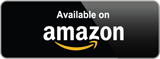# Capacitor Bank calculator or kVAR Calculation Formula

### Capacitor Bank calculator:

Capacitor Bank calculator is used to find the required kVAR for improving power factor from low to high. Enter the current power factor, real power of the system/panel and power factor value to be improved on the system/panel. Then press the calculate button to get the required capacitor bank in kVAR. Reset button shall remove the values in the field.

 Enter Current Powerfactor: Enter Curent Real Power (Load): kW Enter Power Factor to Imporoved: Result Capacitor bank: kVAR

Note: if you want to calculate the capacitor bank in VAR/MVAR means, just enter the real power in W or MW. Example, if you are entering it in kW mean, you get kVAR only. The same way work for W and MW.

### Capacitor Bank calculation

Required reactive power Q(kVR) is equal to the real power P(kW) times of the difference between tangent of cosine inverse of the power factor PF1 to cosine of power factor PF2. The capacitor bank calculator formula can be written as,

Required Reactive Power kVAR = P(kW) x tan (cos-1(PF1)- cos-1(PF2))

Required Reactive Power in VAR = P(W) x tan (cos-1(PF1)- cos-1(PF2))

Required Reactive Power MVAR = P(MW) x tan (cos-1(PF1)- cos-1(PF2))

Example:

A three-phase motor has 100kW real power load at operating at 0.7pf, we need to improve the power factor to 0.96. Let we calculate the required reactive power in kVAR or capacitor bank to be connected across the motor?

Here,

PF1 = 0.7

PF2 = 0.96

Required capacitor bank = 100 x tan (cos-1(0.7)- cos-1(0.96)) = 72.85 kVAR.

Hence you can connect three 25kVAR capacitor bank across the panel for improving the power factor from 0.7 to 0.96

Check the Price for 25kVAR in amazon:1.Mickel Raj

1.capacitor bank for a MSB incoming 1600 amps
2 total motor KW 316KW
3. To improve the PF to 0.9
4. Voltage 415 3ph

1.How many steps capacitor bank is recommended?
2. Whats the KVAR rating capacitor we need to use?
.

•Manimuthu Ayothi

what is your previous power factor?